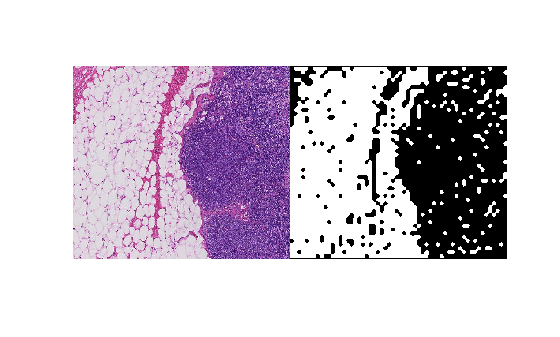# sub2world

Convert pixel subscripts to block subscripts

## Syntax

``world = sub2world(bim,pixelsub)``
``world = sub2world(bim,pixelsub,'Level',L)``

## Description

example

````world = sub2world(bim,pixelsub)` converts the pixel subscripts, `pixelsub`, to the block subscript of the block containing the corresponding pixel. ```
````world = sub2world(bim,pixelsub,'Level',L)` additionally specifies the resolution level to use in a multiresolution image. `Level` defaults to 1.```

## Examples

collapse all

Convert pixel subscripts from one level to another via the world coordinates to refer to the same spatial region.

Create a blocked image from a sample image included with the toolbox.

`bim = blockedImage('tumor_091R.tif');`

Define a region of interest in the finest resolution level in pixel subscripts.

```level1PixelSubStart = [1700, 1550 1]; level1PixelSubEnd = [2100, 2000 3];```

Get the image data from the region of interest on the resolution level 1 image.

```imr = getRegion(bim, level1PixelSubStart, level1PixelSubEnd, "Level", 1); size(imr)```
```ans = 1×3 401 451 3 ```

Convert the pixel subscripts that define the region of interest into world coordinates. By default, `sub2world` converts the coordinates at level 1, the finest resolution.

`worldRegion = sub2world(bim,[level1PixelSubStart; level1PixelSubEnd]);`

Compute a binary mask at the coarsest level.

`bbw = apply(bim, @(bs)imbinarize(im2gray(bs.Data)), "Level", 3);`

Convert the world coordinates of the region of interest to pixel subscripts of the mask. Note that the mask has only two dimensions.

```worldRegion = worldRegion(:,1:2); maskPixelSubs = world2sub(bbw,worldRegion);```

```bwr = getRegion(bbw, maskPixelSubs(1,:), maskPixelSubs(2,:)); size(bwr)```
```ans = 1×2 51 58 ```

View the original image and the mask.

`montage({imr,bwr})`## Input Arguments

collapse all

Blocked image, specified as a `blockedImage` object.

Pixel subscripts, specified as a K-by-N integer-valued vector, where N is the number of dimensions and K is the number of coordinates.

## Output Arguments

collapse all

World subscripts, returned as a K-by-X numeric array. The world coordinates are in the same order as the pixel subscripts. For an N-dimensional `blockedImage`, and K subscripts (each row is a separate subscript), `pixelsub` is a K-by-N integer-valued matrix and `world` is a K-by-N numeric matrix.

## Version History

Introduced in R2021a